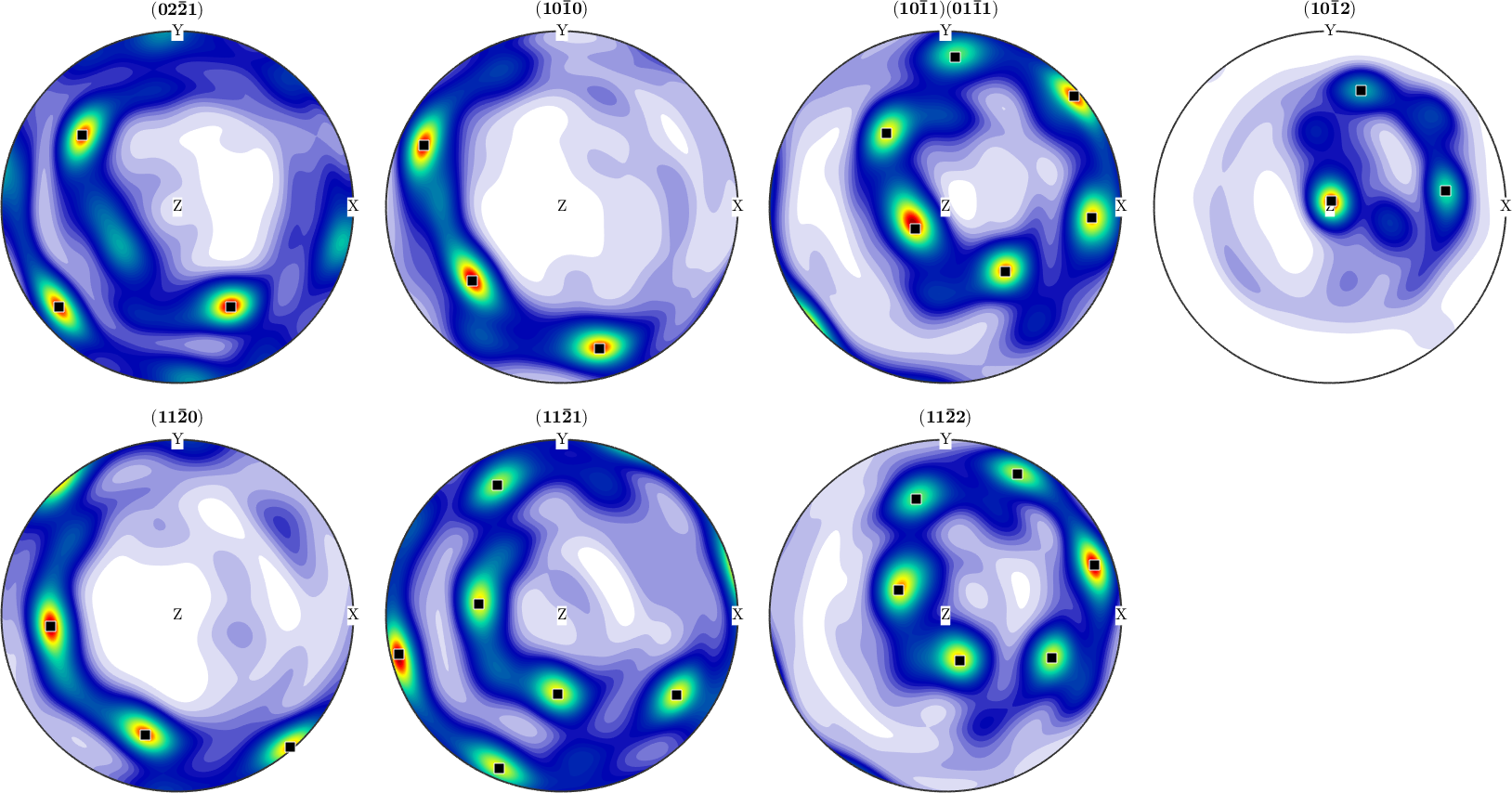ODF Characteristics edit page

Let us first begin with some constructed ODFs to be analyzed below

A bimodal ODF:

A fibre ODF:

An ODF estimated from diffraction data

## Modal Orientations

The modal orientation of an ODF is the crystallographic prefered orientation ori_pref of the texture. It is characterized as the maximum of the ODF. In MTEX it is returned as the second output argument of the command max

Lets mark this prefered orientation in the pole figures## Texture Characteristics

Texture characteristics are used for a rough classification of ODFs into sharp and weak ones. The two most common texture characteristics are the entropy and the texture index. The texture index of an ODF $$f$$ is defined as:

$t = \int_{SO(3)} f({R})^2 dR$

We may either compute this integral using the command sum directly by

or, more efficiently, by the command norm

The entropy of an ODF $$f$$ is defined as:

$H = - \int_{SO(3)} f({R}) \ln f({R}) dR$

## Volume Portions

Volume portions describes the relative volume of crystals having a certain orientation. The relative volume of crystals having a orientation close to a given orientation is computed by the command volume and the relative volume of crystals having a orientation close to a given fibre is computed by the command fibreVolume

The relative volume in percent of crystals with missorientation maximum 30 degree from the preferred orientation ori_pref:

The relative volume of crystals with missorientation maximum 20 degree from the prefered fibre in percent: